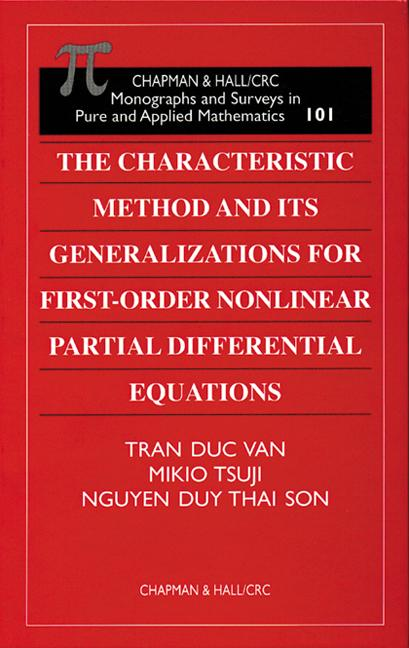# The Characteristic Method and Its Generalizations for First-Order Nonlinear Partial Differential Equations

## 1st Edition

Chapman and Hall/CRC

256 pages

##### Purchasing Options:\$ = USD
Hardback: 9781584880165
pub: 1999-06-25
SAVE ~\$37.00
Currently out of stock
\$185.00
\$148.00
x

FREE Standard Shipping!

### Description

Despite decades of research and progress in the theory of generalized solutions to first-order nonlinear partial differential equations, a gap between the local and the global theories remains: The Cauchy characteristic method yields the local theory of classical solutions. Historically, the global theory has principally depended on the vanishing viscosity method.

The authors of this volume help bridge the gap between the local and global theories by using the characteristic method as a basis for setting a theoretical framework for the study of global generalized solutions. That is, they extend the smooth solutions obtained by the characteristic method.

The authors offer material previously unpublished in book form, including treatments of the life span of classical solutions, the construction of singularities of generalized solutions, new existence and uniqueness theorems on minimax solutions, differential inequalities of Haar type and their application to the uniqueness of global, semi-classical solutions, and Hopf-type explicit formulas for global solutions. These subjects yield interesting relations between purely mathematical theory and the applications of first-order nonlinear PDEs.

The Characteristic Method and Its Generalizations for First-Order Nonlinear Partial Differential Equations represents a comprehensive exposition of the authors' works over the last decade. The book is self-contained and assumes only basic measure theory, topology, and ordinary differential equations as prerequisites. With its innovative approach, new results, and many applications, it will prove valuable to mathematicians, physicists, and engineers and especially interesting to researchers in nonlinear PDEs, differential inequalities, multivalued analysis, differential games, and related topics in applied analysis.

Preface

Local Theory on Partial Differential Equations of First Order

Characteristic Method and Existence of Solution

A Theorem of A. Haar

A Theorem of T. Wazewski

Life Spans of Classical Solutions of Partial Differential Equations of First Order

Introduction

Life Spans of Classical Solutions

Global Existence of Classical Solutions

Behavior of Characteristic Curves and Prolongation of Classical Solutions

Introduction

Examples

Prolongation of Classical Solutions

Sufficient Conditions for Collision of Characteristic Curves I

Sufficient Conditions for Collision of Characteristic Curves II

Equations of Hamilton-Jacobi Type in One Space Dimension

Non-Existence of Classical solutions and Historical Remarks

Construction of Generalized Solutions

Semi-Concavity of Generalized Solutions

Collision of Singularities

Quasi-Linear Partial Differential Equations of First Order

Introduction and Problems

Difference Between Equations of Conservation Law and Equations of Hamilton-Jacobi Type

Construction of Singularities of Weak Solution

Entropy Condition

Construction of Singularities for Hamilton-Jacobi Equations in Two Space Dimensions

Introduction

Construction of Solution

Semi-Concavity of the Solution u = u(t,x)

Collision of Singularities

Equations of Conservation Law without Convexity Condition in One Space Dimension

Introduction

Rarefaction Waves and Contact Discontinuity

An Example of an Equation of Conservation Law

Behavior of the Shock S1

Behavior of the Shock S2

Differential Inequalities of Haar Type

Introduction

A Differential Inequality of Haar Type

Uniqueness of Global Classical Solutions to the Cauchy Problem

Generalizations to the Case of Weakly-Coupled Systems

Hopf's Formulas for Global Solutions of Hamilton-Jacobi Equations

Introduction

The Cauchy Problem with Convex Initial Data

The Case of Nonconvex Initial Data

Equations with Convex Hamiltonians f = f(p)

Hopf-Type Formulas for Global Solutions in the Case of Concave-Convex Hamiltonians

Introduction

Conjugate Concave-Convex Functions

Hopf-Type Formulas

Global Semiclassical Solutions of First-Order Partial Differential Equations

Introduction

Uniqueness of Global Semiclassical Solutions to the Cauchy Problem

Existence Theorems

Minimax Solutions of Partial Differential Equations with Time-Measurable Hamiltonians

Introduction

Definition of Minimax Solutions

Relations with Semiclassical Solutions

Invariance of Definitions

Uniqueness and Existence of Minimax Solutions

The Case of Monotone Systems

Mishmash

Hopf's Formulas and Construction of Global Solutions via Characteristics

Smoothness of Global Solutions

Relationship Between Minimax and Viscosity Solutions

Appendix I: Global Existence of Characteristic Curves

Appendix II: Convex Functions, Multifunction, and Differential Inclusions

References

Index

### Subject Categories

##### BISAC Subject Codes/Headings:
MAT003000
MATHEMATICS / Applied
MAT007000
MATHEMATICS / Differential Equations